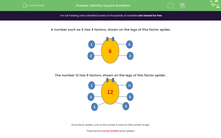# Identify Square Numbers

In this worksheet, children must state whether or not a number is square.Key stage:  KS 2

Curriculum topic:   Number: Multiplication and Division

Curriculum subtopic:   Recognise Square/Cube Numbers

Popular topics:   Square Numbers worksheets, Factors worksheets

Difficulty level:#### Worksheet Overview

Factors are numbers that multiply together to make another number.

For example:

A number such as 6 has 4 factors, shown on the legs of this factor spider.The number 12 has 6 factors, shown on the legs of this factor spider.Some factor spiders, such as the number 9, have an ODD number of legs.

These are the SQUARE NUMBER factor spiders.Remember that 9 = 3 x 3 = 3 squared.

### What is EdPlace?

We're your National Curriculum aligned online education content provider helping each child succeed in English, maths and science from year 1 to GCSE. With an EdPlace account you’ll be able to track and measure progress, helping each child achieve their best. We build confidence and attainment by personalising each child’s learning at a level that suits them.

Get started